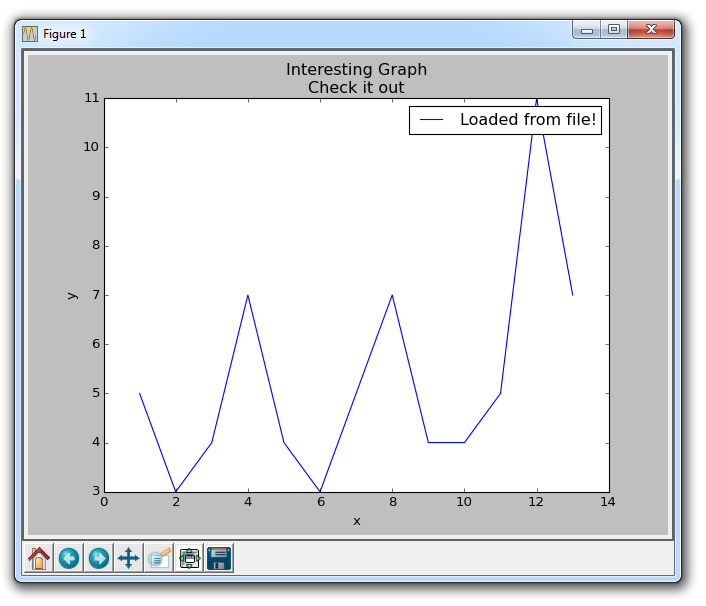# 第七章 从文件加载数据

``````import matplotlib.pyplot as plt
import csv

x = []
y = []

with open('example.txt','r') as csvfile:
for row in plots:
x.append(int(row))
y.append(int(row))

plt.xlabel('x')
plt.ylabel('y')
plt.title('Interesting Graph\nCheck it out')
plt.legend()
plt.show()
````````````1,5
2,3
3,4
4,7
5,4
6,3
7,5
8,7
9,4
10,4
``````

``````import matplotlib.pyplot as plt
import numpy as np

x, y = np.loadtxt('example.txt', delimiter=',', unpack=True)